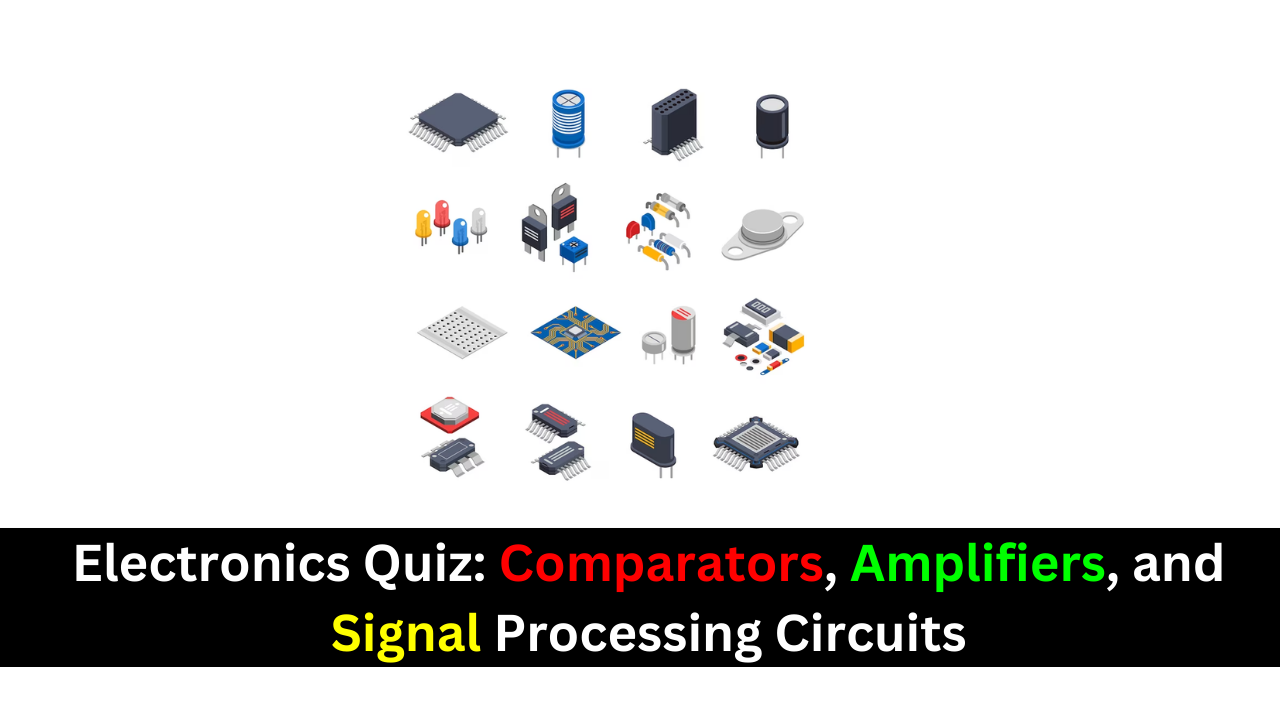Thursday, October 26

## Electronics Quiz: Comparators, Amplifiers, and Signal Processing Circuits### The purpose of a comparator is to

(a) amplify an input voltage
(b) detect the occurrence of a changing input voltage
(c) produce a change in output when an input voltage equals a reference voltage
(d) maintain a constant output when the dc input voltage changes
Answer: (c) produce a change in output when an input voltage equals a reference voltage

### To use a comparator for zero-level detection, the inverting input is connected to

(a) ground
(b) the dc supply voltage
(c) a positive reference voltage
(d) a negative reference voltage

### In a 5 V level detector circuit, the

(a) output is limited to 5 V
(b) inverting input is connected to
(c) input signal is limited to a 5 V peak value
(d) input signal must be riding on a level
Answer: (c) input signal is limited to a 5 V peak value

### To convert a summing amplifier to an averaging amplifier,

(a) all input resistors must be a different value
(b) the ratio must equal the reciprocal of the number of inputs
(c) the ratio must equal the number of inputs
Answer: (b) the ratio must equal the reciprocal of the number of inputs

### In a scaling adder, the

(a) input resistors are all the same value
(b) feedback resistor is equal to the average of the input resistors
(c) input resistors have values that depend on the assigned weight of each input
(d) ratio must be the same for each input
Answer: (c) input resistors have values that depend on the assigned weight of each input

### The feedback path in an ideal op-amp integrator consists of a

(a) resistor (b) capacitor
(c) resistor and a capacitor in series (d) resonant circuit

### The feedback path in an op-amp differentiator consists of a

(a) resistor
(b) capacitor
(c) resistor and a capacitor in series
(d) resistor and a capacitor in parallel
Answer: (c) resistor and a capacitor in series

### The op-amp comparator circuit uses

(a) positive feedback (b) negative feedback
(c) regenerative feedback (d) no feedback

### Unity gain and zero phase shift around the feedback loop are conditions that describe

(a) an active filter (b) a comparator
(c) an oscillator (d) an integrator or differentiator

### To make a basic instrumentation amplifier, it takes

(a) one op-amp with a certain feedback arrangement
(b) two op-amps and seven resistors
(c) three op-amps and seven capacitors
(d) three op-amps and seven resistors
Answer: (d) three op-amps and seven resistors

### Typically, an instrumentation amplifier has an external resistor used for

(a) establishing the input impedance (b) setting the voltage gain
(c) setting the current gain (d) interfacing with an instrument
Answer: (b) setting the voltage gain

### Instrumentation amplifiers are used primarily in

(a) high-noise environments (b) amplitude modulators
(c) test instruments (d) filter circuits

### Isolation amplifiers are used primarily in

(a) remote, isolated locations
(b) systems that isolate a single signal from many different signals
(c) applications where there are high voltages and sensitive equipment
(d) applications where human safety is a concern

### The three parts of a basic isolation amplifier are

(a) amplifier, filter, and power (b) input, output, and coupling
(c) input, output, and power (d) gain, attenuation, and offset
Answer: (c) input, output, and power

### The stages of most isolation amplifiers are connected by

(a) copper strips (b) transformers
(c) microwave links (d) current loops

### The characteristic that allows an isolation amplifier to amplify small signal voltages in the presence of much greater noise voltages is its

(a) CMRR (b) high gain
(c) high input impedance (c) magnetic coupling between input and output

### The term OTA means

(a) operational transistor amplifier (b) operational transformer amplifier
(c) operational transconductance amplifier (d) output transducer amplifier

### In an OTA, the transconductance is controlled by

(a) the dc supply voltage (b) the input signal voltage
(c) the manufacturing process (d) a bias current
Answer: (b) the input signal voltage

### The voltage gain of an OTA circuit is set by

(a) a feedback resistor (b) the transconductance only
(c) the transconductance and the load resistor (d) the bias current and supply voltage

### An OTA is basically a

(a) voltage-to-current amplifier (b) current-to-voltage amplifier
(c) current-to-current amplifier (d) voltage-to-voltage amplifier

### For a given input signal, the minimum output signal voltage of an active positive clamper is

(a) equal to the dc value of the input
(b) equal to the peak value of the input
(c) equal to the voltage connected to the input of the op-amp
(d) equal to the voltage connected to the input of the op-amp
Answer: (c) equal to the voltage connected to the input of the op-amp

### When the input of the clamper op-amp is connected to ground, the dc value of the output voltage is

(a) zero (b) equal to the dc value of the input
(c) equal to the average value of the input (d) equal to the peak value of the input
Answer: (b) equal to the dc value of the input

### In an active positive limiter (similar to the one in Figure 33 except for the direction of the diode) with a sine wave input voltage, the output signal is

(a) limited to values above (b) limited to values below
(c) always 0.7 V (d) like the input signal
Answer: (a) limited to values above

### An active limiter with back-to-back zener diodes across the feedback path limits the

(a) positive peaks only
(b) positive and negative peaks
(c) negative peaks only
(d) positive peak to the zener voltage and the negative peak to -0.7 V
Answer: (b) positive and negative peaks

Related Posts

Share.
error: Content is protected !!